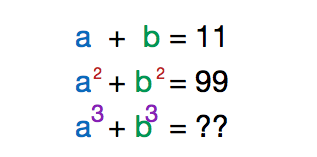# The sum of cubes

Algebra Level 1If the sum of two numbers is 11, and the sum of their squares is 99, find the sum of their cubes.

×